Mathematical and Physical Journal
for High Schools
Issued by the MATFUND Foundation
 Already signed up? New to KöMaL?

# KöMaL Problems in Physics, April 2007

Show/hide problems of signs:## Problems with sign 'M'

Deadline expired on May 11, 2007.

M. 278. Examine experimentally when will the pendulum stop sooner if there is a smaller or a greater bob at the end of the thread? Does the time while the bob stop depend on the material of the bob?

(6 pont)

statistics## Problems with sign 'P'

Deadline expired on May 11, 2007.

P. 3973. Because of the hot weather air-condition was on for about 3 hours every day in July. The rate of energy absorbed from the room is 1800 W, the device is operated from a voltage of 230 V such that the useful rate of energy of the device is 240% of the rate of energy drown from the power supply. How much does the monthly bill increased if the price of 1 kWh energy is 36 Ft.

(3 pont)

statistics

P. 3974. A body of mass m1=2 kg slides down from the top of an inclined plane (the angle of inclination is=20o.) A cart of mass m2=1 kg is attached to this body with a thread of length=1.6 m as shown in the figure. The thread initially is straight. Along the first part of the slope, the length of which is/2, friction is negligible, along the second part, the length of which is/2, the coefficient of friction is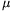=0.6. How far will the two objects be when the cart reaches the pulley?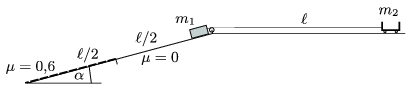(4 pont)

statistics

P. 3975. There are switches at both ends of a long corridor. The lamp in the middle of the corridor can be turned off or on with both switches.

a) Draw the circuit.

b) What fraction of the time is the lamp on if one of the switches is turned at every 2 minutes and the other is switched in every three minutes.

(4 pont)

statistics

P. 3976. A disc of radius R is moved with a constant u velocity on an air-cushioned table. Another small disc collides with it elastically with a speed of v0=0.3 m/s, the velocities of the discs are parallel. The distance d shown in the figure is equal to R/2, friction between the discs is negligible.

a) For which u will the small disc move perpendicularly to its original motion after the collision?

b) What will be the speed of the small disc in this case?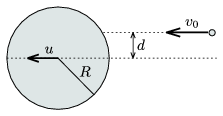(5 pont)

statistics

P. 3977. tional acceleration if the radius of the sphere is 10 km, and the centre of the sphere is 11 km below the surface of the Earth. Calculate the change in the gravitational acceleration exactly above the oil field and 20 km away from this point.

(5 pont)

statistics

P. 3978. A rod of mass m and length=1 m slides down on a slope such that one of its end slides along the slope and the other slides along the horizontal without friction. Initially the rod touches the incline, the figure shows a snapshot of the motion later.

a) Find the speed of the endpoints A and B when the rod reaches the horizontal position.

b) What are these speeds when the rod encloses an angle of 30o with the horizontal.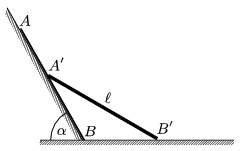(5 pont)

statistics

P. 3979. Two pointlike charges of charge Q and -4Q are fixed at a distance of L.

a) At which point will the electric field be zero.

b) At which point will the net potential be zero?

(5 pont)

statistics

P. 3980. A pair of wire is used to transfer power of 100 kW to a distance of 100 km, such that the decrease in the voltage can be maximum 2%. The wire is made of copper the resistivity of which is 1.7.10-8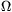m, the diameter of the wire is 20 mm. Calculate the voltage.

(4 pont)

statistics

P. 3981. A generator of constant voltage but of variable frequency is connected to a series RLC circuit. Gradually changing the frequency the current reaches a maximum, when the voltage across the inductor is U1. If the frequency is further increased the current decreases, but the voltage across the inductor still increases. Later the voltage across the coil reaches its maximum of U2 and then it decreases.

Determine the quotient ofin terms of R, L and C.

(5 pont)

statistics

P. 3982. Recently the nuclear technology is appropriate to transfer an element to another. Describe a method how to produce stable lead from stable bismuth.

(5 pont)

statistics